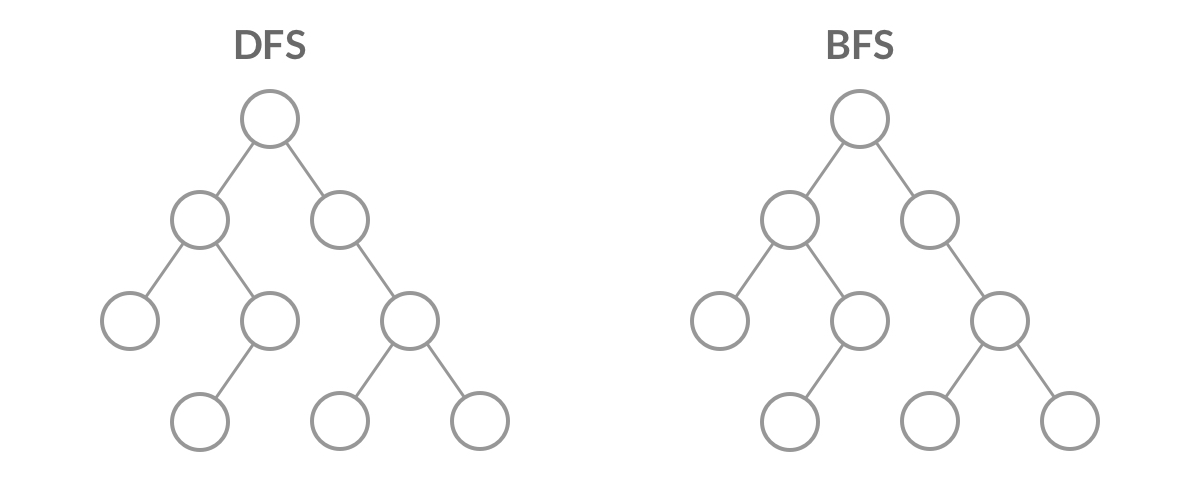﻿ 前端程式員學好演算法系列（六）隊列_ZenDei技術網路在線

# 前端程式員學好演算法系列（六）隊列```二叉樹：[3,9,20,null,null,15,7],

3
/ \
9 20
/ \
15 7```

```[
,
[9,20],
[15,7]
]```1.root為空師返回 []
2.定義隊列為queue，預設在queue中傳入root節點
3.我們記錄一下當前節點的層級i，每次從隊列頭部取出一個節點，如果該節點有左右節點值就把左右節點都重新放入隊列

4.res[res.length-1] 能幫我們取到當前操作的師哪層節點。

```/**
* Definition for a binary tree node.
* function TreeNode(val) {
*     this.val = val;
*     this.left = this.right = null;
* }
*/
/**
* @param {TreeNode} root
* @return {number[][]}
*/
var levelOrder = function(root) {
let res = []
if(root==null){
return []
}
let queue = [root]
while(queue.length){
let len = queue.length
let i = 0
res.push([])
while(i++ < len){
let node = queue.shift()
res[res.length-1].push(node.val)
if(node.left){
queue.push(node.left)
}
if(node.right){
queue.push(node.right)
}
}
}
return res
};```

279. 完全平方數

```輸入: n = 12

```輸入: n = 13

1.創建隊列queue 預設傳入num = n step為0；
2.創建visited 對象記錄 num - i*i 的值是否訪問過，訪問過時無需重覆訪問；
3.操作隊列彈出隊首節點，操作彈出的節點 —— 根據業務生成子節點，判斷這些節點 —— 符合業務條件，則return，不符合業務條件，且不在已訪問集合，則追加到隊尾，並加入已訪問集合

```/**
* @param {number} n
* @return {number}
*/
var numSquares = function(n) {
// 創建一個隊列預設傳入數字num和step 為0
if(n<=1){
return n
}
let queue = [{'num':n,'step':0}]
let visited = {}
visited[n] =true
while(queue.length){
const {num , step} = queue.shift()
if(num==0){
return step
}
for(let i = 1; num - i*i >=0; i++){
if(!visited[num-i*i]){
queue.push({'num':num-i*i,"step":step +1})
visited[num-i*i] = true
}
}
}
};```

```        for(let i = 1; ; i++){
let a = num - i*i
if(a<0) break;
if(a==0) return step +1;
if(!visited[num-i*i]){
queue.push({'num':num-i*i,"step":step +1})
visited[num-i*i] = true
}
}```

347. 前 K 個高頻元素

```輸入: nums = [1,1,1,2,2,3], k = 2

```輸入: nums = , k = 1

1.遍歷一遍數組統計每個元素的數量
2.定義priority_queue 保存 [元素的頻率，元素的值]
3.排序數組（這裡應該用最小堆進行排序）
4.建立res 獲取到需要的值

```/**
* @param {number[]} nums
* @param {number} k
* @return {number[]}
*/
var topKFrequent = function(nums, k) {
let obj = {}
for(let i =0;i<nums.length;i++){
if(!obj[nums[i]]){
obj[nums[i]] = 1
} else {
obj[nums[i]] = obj[nums[i]] + 1
}
}
// priority_queue 保存的內容為[元素的頻率,元素值]
let priority_queue = []
for(let i in obj){
// if(priority_queue.length==k){
priority_queue.push([obj[i],i])
//  }
}
if(priority_queue.length==1){
return [priority_queue]
}
priority_queue.sort((a,b)=>b-a)

let res= []
for(let i =0;i<priority_queue.length;i++){
if(res.length<k){
res.push(priority_queue[i])
}else {
return res
}
}
return res

};```• 作者：吉玉 智能化測試 在互動中經常需要維護大量的狀態，對這些狀態進行測試驗證成本較高，尤其是當有功能變動需要回歸測試的時候。為了降低開發測試的成本，在這方面使用強化學習模擬用戶行為，在兩個方面提效： mock介面：將學習過程中的狀態作為服務介面的測試數據； 回歸測試：根據mock介面數據回溯到特定 ...
• 144. 二叉樹的前序遍歷給定一個二叉樹，返回它的 前序 遍歷。 示例: 輸入: [1,null,2,3] 1 \ 2 / 3 輸出: [1,2,3] 有兩種通用的遍歷樹的策略： 深度優先搜索（DFS） 在這個策略中，我們採用深度作為優先順序，以便從跟開始一直到達某個確定的葉子，然後再返回根到達另一個 ...
• 當我們使用了websocket技術實現了實時的推送訪客來到消息,但是我們只能隨時看著頁面不離開才能知道有訪客來到 現在增加瀏覽器的通知消息,就可以只要打開著界面,不用一直盯著,乾別的事去就可以了,有新消息會從右下角彈出來 測試地址如下,當有訪客點擊咨詢按鈕時,客服後臺會彈出提示: https://g ...
• 1.驗證ip地址 <input name="ip" class="mini-textbox" required emptyText="請輸入ip地址" onvalidation="isIpAddress" /> // ip地址驗證 function isIpAddress(e){ var reg = ...
• 在日常佈局當中，肯定經常會遇到文本內容超過容器的情況。非常常見的一種解決方案是超出省略。 但是，有的時候，由於場景的限制，可能會出現在一些無法使用超出打點省略的方法的場景，譬如在導航欄中： 這種情況下，在容器定寬但是文本又溢出且不能換行的情況下，我們就需要尋求另外的解決方案。 hover 時彈出框提 ...
• 在接觸 Web前端開發的一段時間，有時會去看Google或者百度的源代碼，有某些地方定義了 data-key ,這種語法 但是如果你直接去 Google data-key 或 data-item 可能又查不到這個屬性，到底這是哪個屬性呢？ 通過查資料我發現，利用 HTML 5，可以為元素添加data ...
• 1.js 前端將平級數據轉為樹形數據的方法 轉，實測成功 https://segmentfault.com/a/1190000022444269 // 將同級數據轉化為樹形結構 listToTree(dataSource) { // datasource是平級的數據來源 return dataSou ...
• 給出一個 32 位的有符號整數，你需要將這個整數中每位上的數字進行反轉 示例 1: 輸入: 123 輸出: 321 示例 2: 輸入: -123 輸出: -321 示例 3: 輸入: 120 輸出: 21 /** * @param {number} x * @return {number} */ v ...